## Bulk Modulus for Gases

Gases have an equation of state that connects pressure, density and temperature i.e., equation of state. This enables us to deduce an explicit relationship between pressure and density. Such a relationship depends upon the process being considered - isothermal (at constant temperature) or isentropic (at constant entropy). The deduced equations are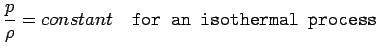(1.20)

and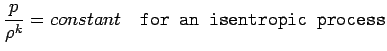(1.21)

whereis the ratio of specific heat at constant pressure,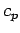to the specific heat at constant volume,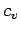. In addition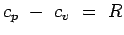, where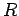is the gas constant. Under ordinary conditions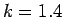for air.

By substituting equations 1.20 and 1.21 in the definition for bulk modulus, Eqn. 1.19 we have,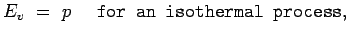(1.22)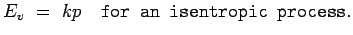(1.23)

Thus we see that the bulk modulus for a gas depends upon its pressure. Given that for air the atmospheric pressure at STP conditions is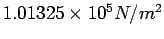, the bulk modulus is of the same order ( while that for water it is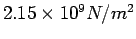). These figures show that air is about 15,000 times as compressible as water.

(c) Aerospace, Mechanical & Mechatronic Engg. 2005
University of Sydney